NCERT Exemplar - MCQs

Chapter 5 Class 12 Continuity and Differentiability
Serial order wise

## (D) none of these

This question is similar to Ex 5.1, 19 - Chapter 5 Class 12 - Continuity and Differentiability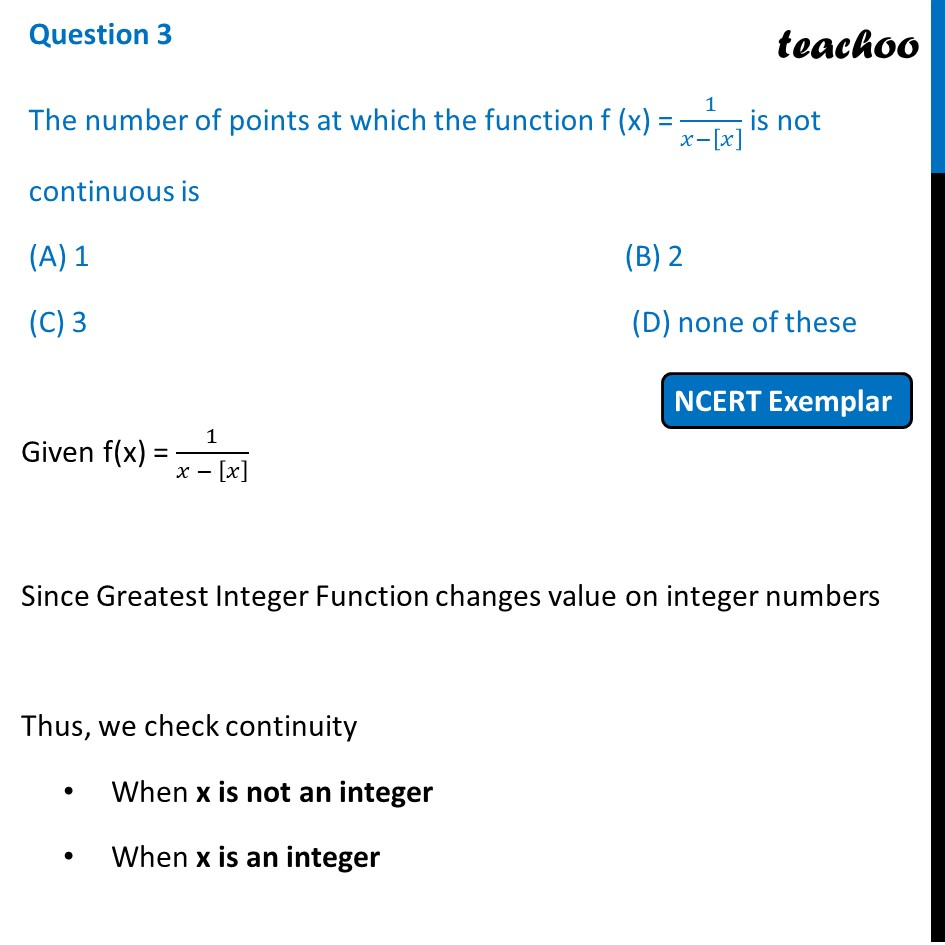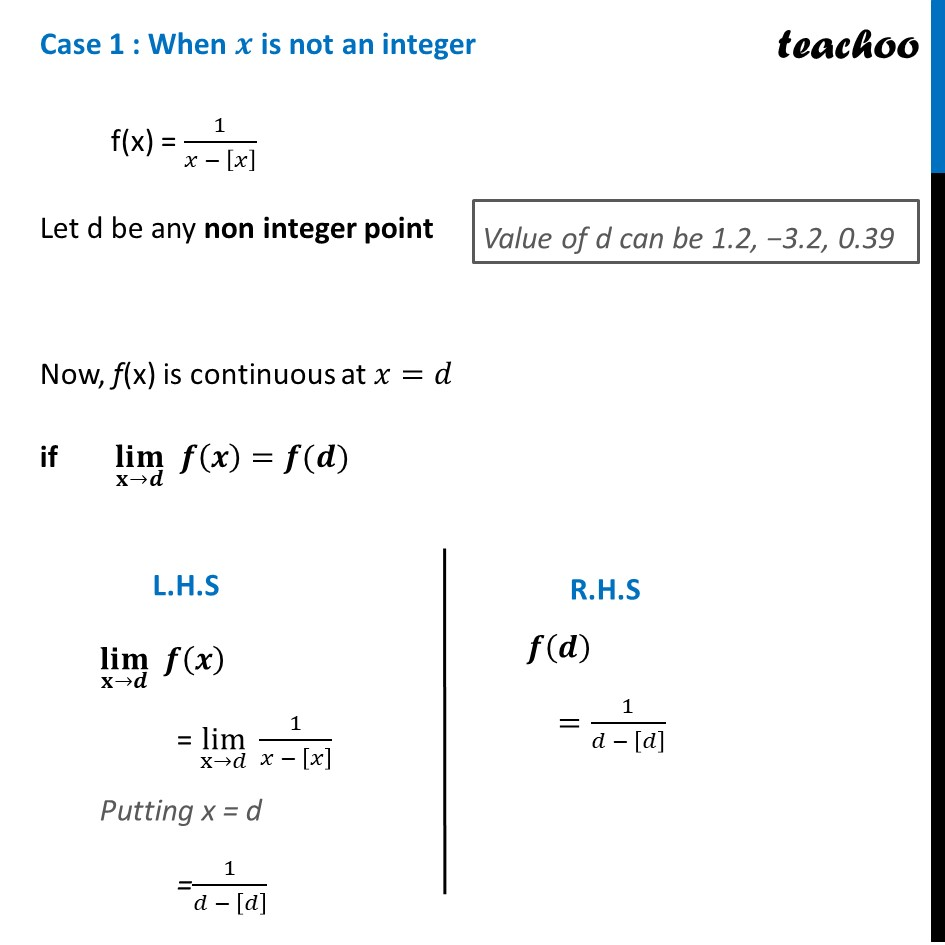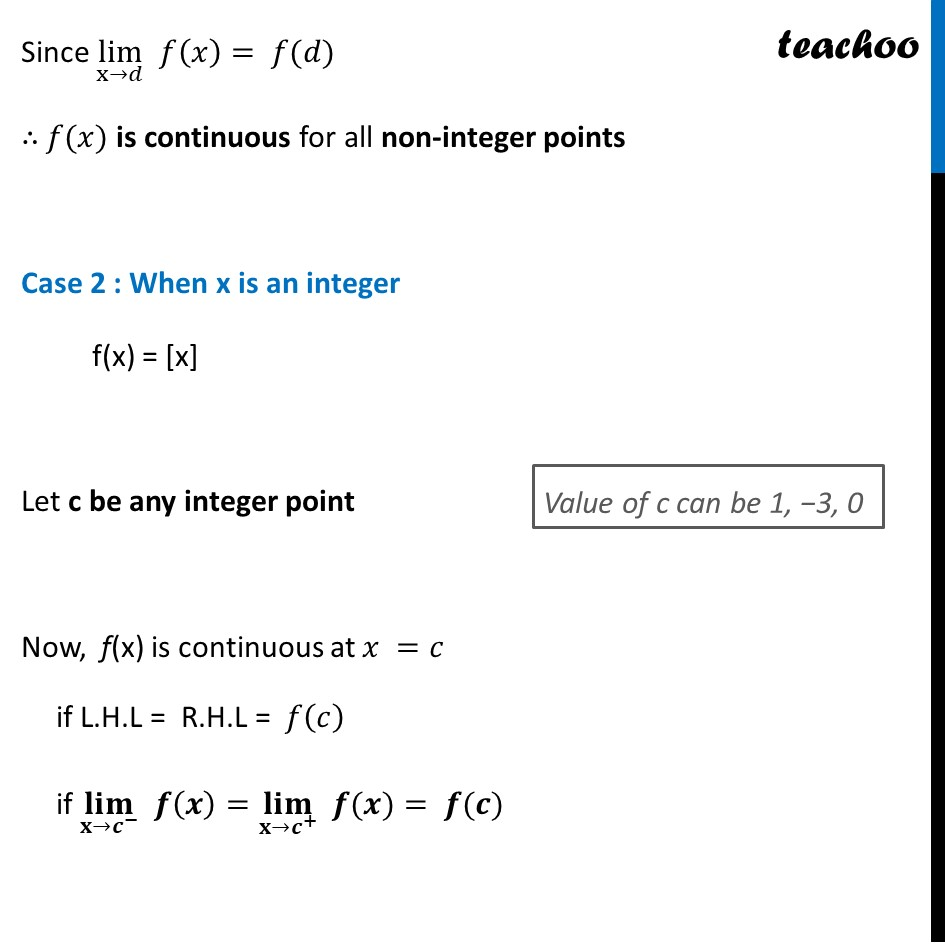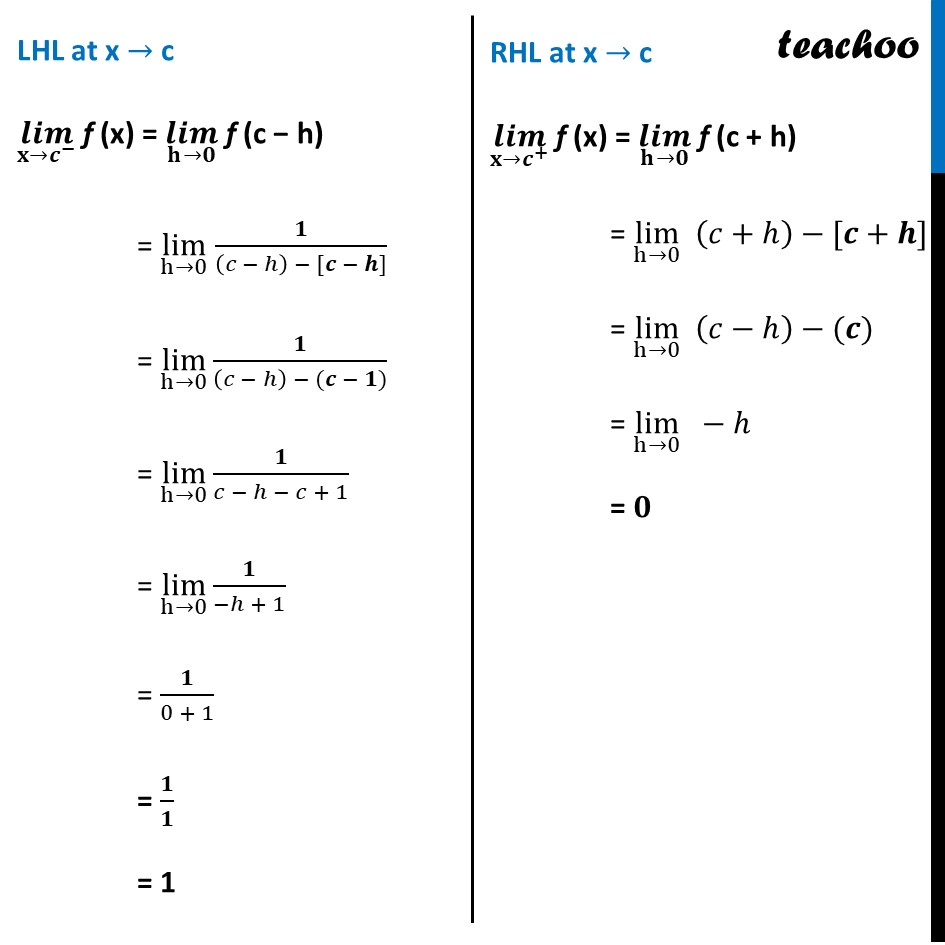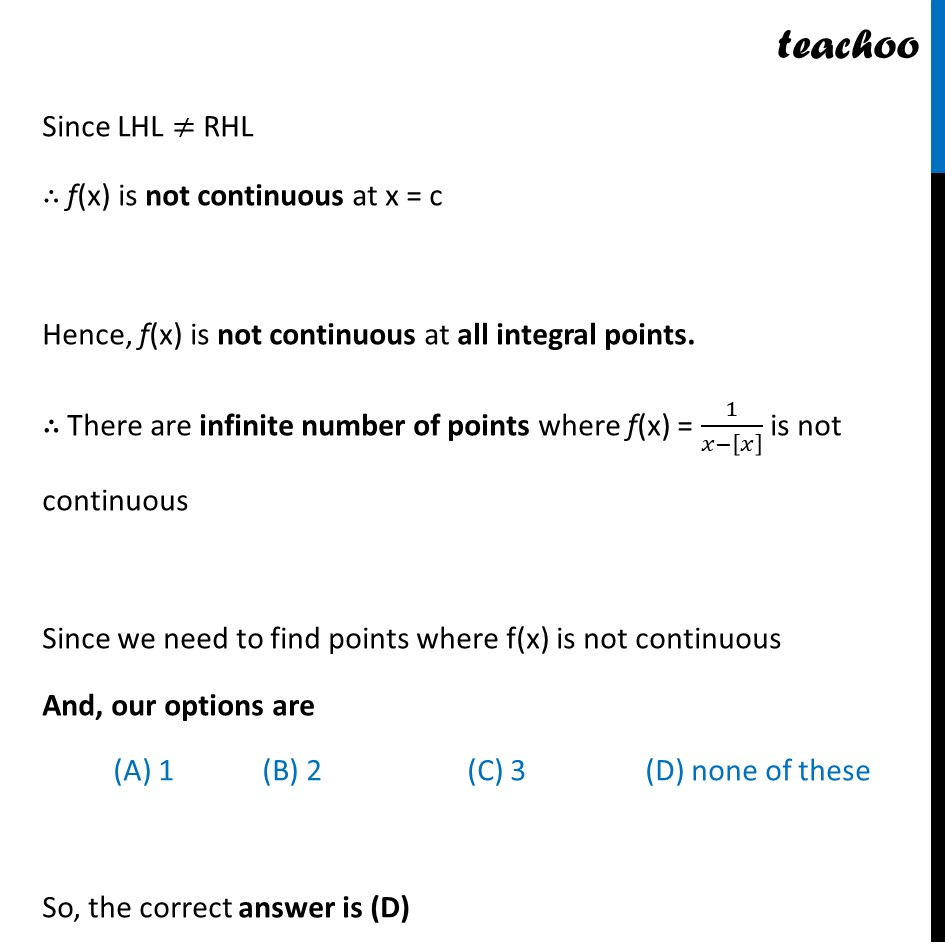Learn in your speed, with individual attention - Teachoo Maths 1-on-1 Class

### Transcript

Question 3 The number of points at which the function f (x) = 1/(𝑥−[𝑥] ) is not continuous is (A) 1 (B) 2 (C) 3 (D) none of these Given f(x) = 1/(𝑥 − [𝑥] ) Since Greatest Integer Function changes value on integer numbers Thus, we check continuity When x is not an integer When x is an integer Case 1 : When 𝒙 is not an integer f(x) = 1/(𝑥 − [𝑥] ) Let d be any non integer point Now, f(x) is continuous at 𝑥=𝑑 if (𝐥𝐢𝐦)┬(𝐱→𝒅) 𝒇(𝒙)=𝒇(𝒅) (𝐥𝐢𝐦)┬(𝐱→𝒅) 𝒇(𝒙) = lim┬(x→𝑑) 1/(𝑥 − [𝑥] ) Putting x = d =1/(𝑑 − [𝑑] ) 𝒇(𝒅) =1/(𝑑 − [𝑑] ) Since lim┬(x→𝑑) 𝑓(𝑥)= 𝑓(𝑑) ∴ 𝑓(𝑥) is continuous for all non-integer points Case 2 : When x is an integer f(x) = [x] Let c be any integer point Now, f(x) is continuous at 𝑥 =𝑐 if L.H.L = R.H.L = 𝑓(𝑐) if (𝐥𝐢𝐦)┬(𝐱→𝒄^− ) 𝒇(𝒙)=(𝐥𝐢𝐦)┬(𝐱→𝒄^+ ) " " 𝒇(𝒙)= 𝒇(𝒄) LHL at x → c (𝒍𝒊𝒎)┬(𝐱→𝒄^− ) f (x) = (𝒍𝒊𝒎)┬(𝐡→𝟎) f (c − h) = lim┬(h→0) 𝟏/((𝑐 − ℎ) − [𝒄 − 𝒉]) = lim┬(h→0) 𝟏/((𝑐 − ℎ) − (𝒄 − 𝟏)) = lim┬(h→0) 𝟏/(𝑐 − ℎ − 𝑐 + 1) = lim┬(h→0) 𝟏/(−ℎ + 1) = 𝟏/(0 + 1) = 𝟏/𝟏 = 1 RHL at x → c (𝒍𝒊𝒎)┬(𝐱→𝒄^+ ) f (x) = (𝒍𝒊𝒎)┬(𝐡→𝟎) f (c + h) = lim┬(h→0) (𝑐+ℎ)−[𝒄+𝒉] = lim┬(h→0) (𝑐−ℎ)−(𝒄) = lim┬(h→0) −ℎ = 𝟎 Since LHL ≠ RHL ∴ f(x) is not continuous at x = c Hence, f(x) is not continuous at all integral points. ∴ There are infinite number of points where f(x) = 1/(𝑥−[𝑥] ) is not continuous Since we need to find points where f(x) is not continuous And, our options are (A) 1 (B) 2 (C) 3 (D) none of these So, the correct answer is (D)